﻿ Common Core Standards for Math - KindergartenAdaptive Worksheets

Helping Every Child to Succeed

toll-free (888) 777-0876
fax (888) 777-0875

Third Grade

## Number and Operations in Base Ten - Fractions

#### Develop understanding of fractions as numbers

3.NF.A.1

Understand a fraction 1/b as the quantity formed by 1 part when a whole is partitioned into b equal parts; understand a fraction a/b as the quantity formed by a parts of size 1/b.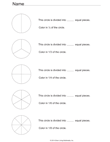Fractions of A Circle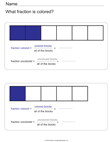Fractions of A Rectangle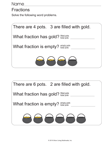St. Patrick's Day Fractions

3.NF.A.2

Understand a fraction as a number on the number line; represent fractions on a number line diagram.

3.NF.A.2.A
Represent a fraction 1/b on a number line diagram by defining the interval from 0 to 1 as the whole and partitioning it into b equal parts. Recognize that each part has size 1/b and that the endpoint of the part based at 0 locates the number 1/b on the number line.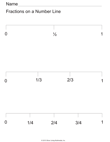Fractions On A Number Line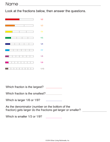Compare Fractions

3.NF.A.2.B
Represent a fraction a/b on a number line diagram by marking off a lengths 1/b from 0. Recognize that the resulting interval has size a/b and that its endpoint locates the number a/b on the number line.

3.NF.A.3

Explain equivalence of fractions in special cases, and compare fractions by reasoning about their size.

3.NF.A.3.A
Understand two fractions as equivalent (equal) if they are the same size, or the same point on a number line.

3.NF.A.3.
B
Recognize and generate simple equivalent fractions, e.g., 1/2 = 2/4, 4/6 = 2/3. Explain why the fractions are equivalent, e.g., by using a visual fraction model.

3.NF.A.3.C
Express whole numbers as fractions, and recognize fractions that are equivalent to whole numbers. Examples: Express 3 in the form 3 = 3/1; recognize that 6/1 = 6; locate 4/4 and 1 at the same point of a number line diagram.
3.NF.A.3.D
Compare two fractions with the same numerator or the same denominator by reasoning about their size.  Recognize that comparisons are valid only when the two fractions refer to the same whole.  Record the results of comparisons with the symbols >, =, or <, and justify the conclusions, e.g., by using a visual fraction model.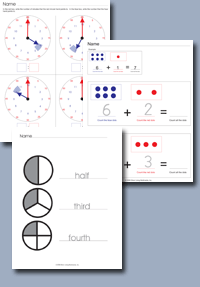Click on any of the standards on the left to create custom worksheets or to print premade materials.